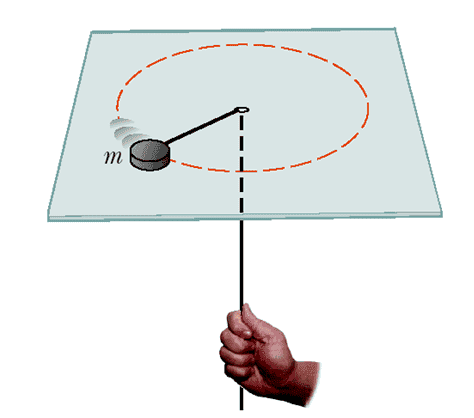# Angular Momentum: Puck Spinning

• minimario

## Homework Statement

The puck in the figure shown below has a mass of 0.120 kg. Its original distance from the center of rotation is 40.0 cm, and it moves with a speed of 80.0 cm/s. The string is pulled downward 15.0 cm through the hole in the frictionless table. Determine the work done on the puck.## Homework Equations

## L = I \omega##
##KE = 1/2 \cdot I \cdot \omega^2 ##

## The Attempt at a Solution

Consider angular momentum. Note it's conserved.

Therefore, ## \frac{1}{2}mr_i^2 \omega_i = \frac{1}{2} mr_f^2 \omega_f \Rightarrow r_i^2 \omega_i =r_f^2 \omega_f ##

Note that ##\omega_i = 80 cm/s = 2 rad/s## (because 2pi radians is one revolution, which is 80pi cm, so 1 radian is 40 cm)

Threrefore, ##0.40^2 \cdot 2 = 0.25^2 \cdot \omega_f \Rightarrow \omega_f = 5.12##

Note that Work Done is the change in kinetic energy. KE initial is equal to ## 1/2 (1/2 \cdot 0.12 \cdot 0.4*0.4) \cdot 2^2 ## (1/2 Iw^2 = 1/2 (1/2mr^2)w^2). KE final is simialrly equal to ## 1/2 (1/2 \cdot 0.12 \cdot 0.25 \cdot 0.25 \cdot 5.12^2##.

We can do the calculation, to get the change as 0.029952. But the book says answer is 5.99*10^-2.

What's wrong?

KE initial is equal to ## 1/2 (1/2 \cdot 0.12 \cdot 0.4*0.4) \cdot 2^2 ## (1/2 Iw^2 = 1/2 (1/2mr^2)w^2). KE final is simialrly equal to ## 1/2 (1/2 \cdot 0.12 \cdot 0.25 \cdot 0.25 \cdot 5.12^2##.
What's the (extra) factor of 1/2 for? (I see an extra one in both initial and final KE's)

You don't even need to bother with angular velocities. (You can solve it that way if you'd like, but it's redundant.) You can just use the angular momentum of a particle = mvr

##KE = 1/2 \cdot I \cdot \omega^2 ##

##\ldots##

Therefore, ## \frac{1}{2}mr_i^2 \omega_i = \frac{1}{2} mr_f^2 \omega_f \Rightarrow r_i^2 \omega_i =r_f^2 \omega_f ##

Do you see the discrepancy between the two quoted lines?

Last edited:
What's the (extra) factor of 1/2 for? (I see an extra one in both initial and final KE's)

You don't even need to bother with angular velocities. (You can solve it that way if you'd like, but it's redundant.) You can just use the angular momentum of a particle = mvr

How do you get that angular momentum of a particle = mvr

@tms, EDIT: No, the kinetic energy isn't being calculated there. That's the conservation of angular momentum, which is Iw.

FINAL EDIT: I see the problem now. Moment of inertia is mr^2, not 1/2 mr^2.

How do you get that angular momentum of a particle = mvr
Angular momentum of a particle equals Iω, but I=mr2, so the angular momentum is mωr2
Since, ωr=v, the angular velocity is mvr

Angular momentum of a particle equals Iω, but I=mr2, so the angular momentum is mωr2
Since, ωr=v, the angular velocity is mvr
You mean, the angular momentum is mvr, yes?

You mean, the angular momentum is mvr, yes?
Oops! Mindless typo, thanks.